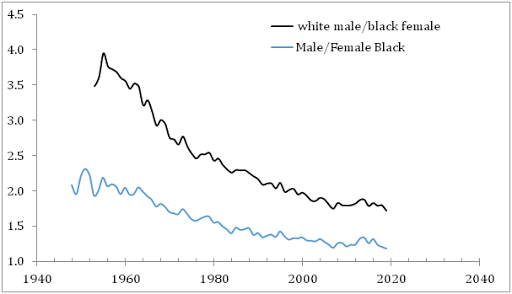## 9/13/11

### On the evolution of age dependent mean income

I published a dozen papers on personal income distribution between 2003 and 2009. One of the principal topics was the evolution of the mean (median) income and its dependence on age. Specifically,  I have shown that the age of largest mean income increases proportionally to the square root from real GDP per capita. (Actually, the mean income was used instead of GDP per capita because the Census Bureau does not include several important sources in the Current Population Surveys. See Figure 1 for differences.) Using this link I have also predicted that this peak mean income will enter the age group between 55 and 64 years after 2015.  Before 1975, the peak mean income was in the age group between 35 and 44 years. Figure 2 presents the mean incomes in various age groups as normalized to the largest mean income in a given year.
According to Figure 1, the growth in the mean income practically stopped in 2000 and the real GDP per capita has returned to 2004. Accordingly, the growth in the age of the largest mean income also effectively stopped in 2004. Since we do not expect fast economic growth in the 2010s, the age of peak mean income may move in the 55 to 64 year group around 2020.
This observation is a crucial one for our model of personal income distribution. It is the only model which ties the age dependent income distribution with the level of real GDP per capita. Slow economic growth is equivalent to slow evolution of personal income distribution. At the same time, the age dependent personal income distribution does not depend on other factors: calendar time, education, human capital, taxes, interest rate, etc.
Figure 1. Real GDP per capita (measured in chained 2009 \$) and mean income ( in 2010 \$) between 1967 and 2010. The mean income is related only to people with income (211,000,000 in 2010) and the GDP per capita is calculated for the whole population.
Figure 2. Mean incomes in various age groups (“20” corresponds to the ages between 15 and 24, and so on) normalized to the largest mean income for a given year. For example, the largest mean income in 2010 belongs to the age group between 45 and 54 years and thus the normalized mean income is 1.0 for this group.

### The mean income gap between white males and black females grows during the democratic presidencies

Two days ago, we compared the mean income evolution of the white and black population and demonstrated that the difference did not change mu...